# E-learning in analysis of genomic and proteomic data 2. Data analysis2.2. Analysis of high-density genomic data2.2.1. DNA microarrays2.2.1.8. Analysis of arrayCGH

Methods based on Hidden Markov models (HMM), where hidden states represent copy numbers relative to the groups of clones, are a compromise between segmentation and clustering approaches. The HMM approach to arrayCGH data has first been suggested by Fridlyand et al. , proposing an algorithm based on discrete states. A discrete HMM model for arrayCGH data with continuous output is characterized as follows (based on  and ):

(1) By the number of states in the model K. These states are hidden and, generally, physically meaningful (e.g. deletion, normal, amplification). Typically, the states are interconnected in such a way that any state can be reached from any other state. We denote the individual states as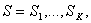and the state at the location l as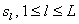.

(2) By the initial state distribution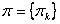, where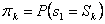,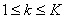.

(3) By the state transition probability distribution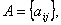where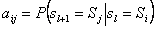,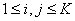For the special case of a model in which all states are connected,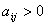for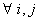.

(4) By the emission distribution or state observation probability density function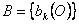where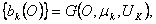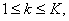where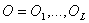is the vector of observed values being modeled, and G is Gaussian density with mean vector mk and covariance matrix Uk.

The parameters of the model are usually denoted by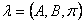.

In the arrayCGH framework, the vector of observed log2ratios represents O and the states are represented by hidden copy numbers respective to groups of clones. When fitting the K-state model on O, the forward-backward algorithm is used to estimate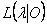- the likelihood of parameters l given the vector O. Then, the optimal state sequence associated with the given sequence of observations O is estimated by the Viterbi algorithm. For each observation oi, the individually most likely state si is selected. Finally, the Baum-Welch (or Expectation-Maximization) algorithm is applied to re-estimate parameters of the model by maximizingThis is the main principle of the HMM approach described in  but there are several other approaches based on HMM models. They differ in the model definition, the algorithms used for fitting of the model, and in the specification of initial parameters.

In Fridlyand et al. , the vector of initial probabilities p is fixed by placing a majority of the weight on the state corresponding to “normal” (or median of log2ratios for a given sample) and the remaining probability is distributed uniformly among all other states (copy number changes). To initialize A, a high probability is assigned to remaining in the same state and low non-zero probabilities to transitions between states. The initial parameters of the emission distributions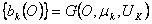are estimated by segmenting the observations in K states using partitioning among medoids (PAM procedure, ) and estimating the mean for each state by the median of the log2ratios of the clones that were allocated to that state. The initial variance is considered to be common and fixed and is estimated similarly. The optimal number K of states is estimated via minimization of the penalized function, based on Akaike information criterion (AIC; ) or BIC (Bayesian information criterion, ). For the final model, a threshold based-approach is used to cluster the estimated K states into biologically relevant states. This approach calculates the median for each state and identifies the two states of which the medians are the closest by computing distance d. If the distance is smaller than the threshold value, these two states are merged and the process is repeated while the condition d<threshold holds true. Thus, only two main parameters are to be set by user: Kmax, the maximal K to be inspected, and the threshold. Fridlyand et al.  report that Kmax=5 is usually sufficient even for very complicated tumor profiles. The choice of threshold is rather dependent on the specific aim of the study and desired type I­ and type II error rate.

As mentioned above, several other HMM-based procedures have been put forward so far. Marioni et al.  proposed a BioHMM procedure that incorporates real distances between clones. This is achieved by allowing transition probabilities that depend on the distance between clones. This means that the Markov chain is no more homogenous and a transition probability matrix Al is defined for each of l-1 transitions between adjacent clones.  report better results in comparison to HMM proposed in , especially for chromosomes where some regions are densely covered and others are covered at lower resolution. However, Stjernqvist et al.  point out that for that definition of transition matrix the Chapman-Kolmogorov equation A(t1)A(t2) = A(t1 + t2), where A(t) is a transition probability matrix between two clones separated by distance t, does not hold. Because of that a Markov chain with the BioHMM transition probabilities does not exist. Moreover they put forward a continuous-index HMM as another solution for designs with unevenly spaced clones which also makes the analysis of overlapping clones feasible. This model computes with transition rates rather than with transition probabilities and allows the state change occur at any base pair position (even in the middle of a clone). The number of states in their model is determined as in the discrete-index HMMs, and for parameter estimation, the Monte Carlo EM (MCEM) algorithm is used. Finally, the realizations of the Markov chain are generated using a number of Markov Chain Monte Carlo (MCMC) simulations.

The above mentioned HMM-based approaches estimate the number of hidden states K via model selection and perform a separate analysis for each chromosome. Such approaches may easily overfit the model parameters to local effects in the chromosomes. Moreover, similarly to some segmentation methods, post-processing by clustering for the detection of biologically relevant labels (deletion, normal, amplification, etc.) is needed as the chosen K has no intrinsic biological meaning.

An approach specifically dealing with this problem was proposed by Guha et al. . It is based on a Bayesian HMM model that uses information priors to specify parameters of the emission distribution. Guha et al. assume a model of K=4 states, where S1 corresponds to single copy loss­, S2 corresponds to normal, S3 to single copy gain, and S4 to multiple copy gain. This leads to the ordering m1< m2< m3< m4 of means of respective state observation probability density functions. According to these assumptions, interval restrictions are put on the parameters of the emission distribution, based on theoretical values. This allows simultaneous segmentation and clustering applying the Viterbi algorithm. Shah et al.  extended this model in two ways, by adding a procedure to improve the robustness against outliers, and by allowing for location-specific priors (LSPs), which can be used to encode known locations of copy number polymorphisms (CNPs). This is achieved by replacing the Gaussian observation model with a mixture of Gaussians with two mixture components. One component represents the log2ratio expected from the given state (loss, normal or gain), the other the log2ratio expected from an outlier.

Rueda & Díaz-Uriarte  believe that an appropriate method for arrayCGH analysis should consider real distances between clones, should provide probabilities of a copy number change instead of p-values or region means, and, depending on the focus of study, should allow for either chromosome by chromosome or genome-wide analysis. They propose a reversible jump aCGH (RJaCGH) method that fulfills these requirements and is applicable to a wide range of aCGH platforms. The method fits a non-homogeneous HMM via reversible jump MCMC. For the final estimate of the number of states, the Bayesian model averaging is used to account for model uncertainty rather than an explicit setting (i.e. number of states in the models) or a selection applying a penalty.  report better performance over several other approaches and conclude that the relative advantage of RJaCGH increases with increasing interprobe variability and noise in the data. Comparable to , the transition probabilities are dependent on the distance between clones; furthermore, the probability of remaining in the same hidden state is a decreasing function of the distance between a probe and a previous probe. When the distance between two clones is maximal, the state of a probe should not be affected by the state of the previous clone. However, this method does not incorporate overlap between clones.

One of the last approaches which in a similar fashion to Stejrnqvist et al. (2007) incorporate both real distance and overlap between clones, was most recently suggested by Andersson . The method is called Segmental Maximum A Posteriori (SMAP) and is also based on a discrete-state HMM model. Like the approach of Guha et al. , it incorporates prior information about the possible source of noise into the emission distribution. The distance-based transition matrix is defined as in , probabilities of possible transition events between two clones should converge towards equality when their positions are genomically distant. To account for clone overlap, changes in intensity ratio are weighted against genomic overlap of previous clones. Segments of clones with deviations in intensity ratio are ignored if the overlap of clones at a previous state is sufficiently prominent. The number of states remains to be defined by the user, however, similarly to , Andersson et al.  recommend to use the six-state model corresponding to double copy loss, one copy loss, normal, one copy gain, double copy gain and multiple copy gain. The main difference (in terms of efficacy of detecting breakpoints) between their approach and the continuous approach due to  is that SMAP does not suffer from exorbitant computational time demand. Also most recently, another kind of Bayesian HMM model was proposed in . Their model is a fitted in three states and incorporates distances between clones. Posterior probabilities are used for the final assessment of segments.